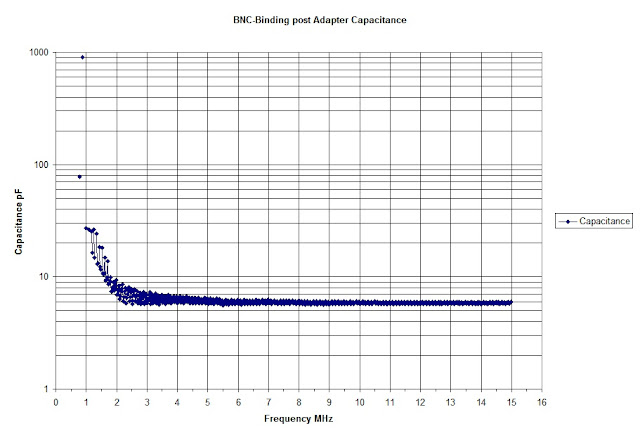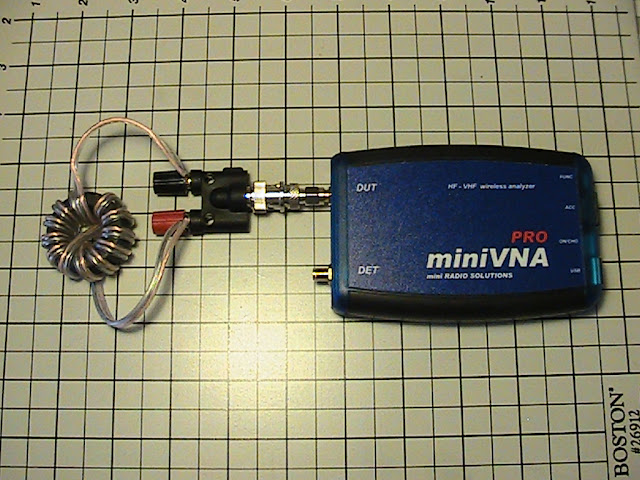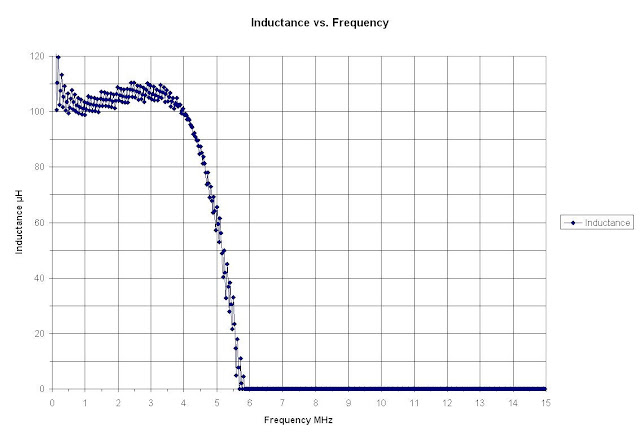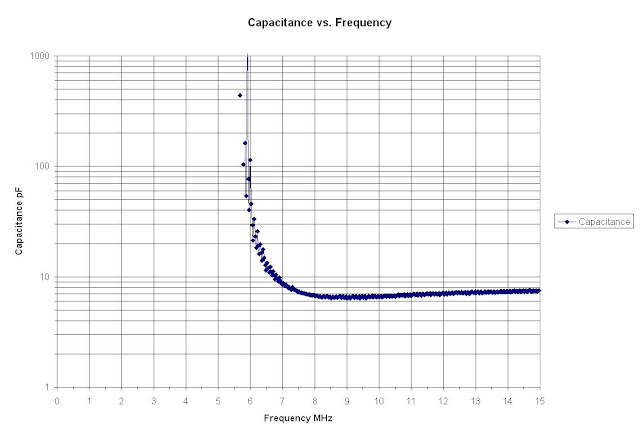# Calculating Unknown Capacitance and Inductance with miniVNA

## Method using Spreadsheet Data exported from the miniVNA Vector Network Analyzer

by Dr. Carol F. Milazzo, KP4MD (posted 10 August 2013)
E-mail: [email protected]

When calibrated with an open load on the DUT port, the miniVNA data output should be compensated for its internal parasitic capacitance.  However, one must determine the additional capacitance of the attached connectors and adapters to accurately calculate the capacitance or inductance of a component attached to the DUT port in reflection mode.  Here is a description of a procedure I followed:

After calibrating the miniVNA Pro per instructions, I attached my Pan Pacific RFA-8382 SMA to BNC and Pomona Electronics model 1296 BNC male to binding post adapter to the DUT port and performed a scan in reflection mode from 0-15 MHz. After exporting the data to an Excel spreadsheet, I added the values below into cells K2 through N3 to the right of the SWR column "J" in order to calculate the capacitance or inductance and Q factor from the measured reactance and resistance using the formulas L=Xl/(2πf), C=-1/(2πfXc), and Q=X/R

K
L
M
N
2
Frequency MHz Capacitance pF Inductance µH Q factor
3
=A3*0.000001 IF(G3<0,1/(2*PI()*A3*-G3)*1000000000000,"") =IF(G3>0,G3/(2*PI()*A3)*1000000,"") =ABS(G3)/F3

I then copied K3, L3, M3 and N3 and filled them to all the cells below them in the rows that are populated with data. Here is the X-Y scatter chart of the data in columns K and L showing capacitance versus frequencyA miniVNA scan with the Pan Pacific RFA-8382 SMA to BNC and Pomona Electronics 1296 BNC to binding post adapters connected to the DUT port yields 5.85 pF calculated mean capacitance over 13-15 MHz.

The calculated capacitance at higher frequencies shows least variance, and the mean capacitance over 13 to 15 MHz is 5.85 pF. This is the parasitic capacitance to be subtracted from unknown loads measured through these adapters.

I then connected a toroid inductor of 13 turns of 18AWG zipcord wound on an FT140-43 toroid core to the miniVNA through the adapters.13 turns of 18AWG speaker wire on an FT140-43 toroid core inductor under test with miniVNA Pro via a Pan Pacific RFA-8382 SMA to BNC adapter and a Pomona 1296 BNC to binding post adapter.

A scan of the toroid inductor from 0-15 MHz was exported to another Excel spreadsheet and the values for inductance and capacitance were calculated as explained above, yielding the following data:A miniVNA scan of the 13 turns of 18AWG speaker wire on FT140-43 toroid core yields 105 µH calculated mean inductance over 1-3 MHz.A miniVNA scan of the 13 turns of 18AWG speaker wire on FT140-43 toroid core yields 7.35 pF calculated mean capacitance over 13-15 MHz.

Of the 7.35 pF calculated mean capacitance over 13-15 MHz, 1.5 pF represents the parasitic capacitance of the inductor and 5.85 pF is the capacitance of the adapters connected to the DUT port.
The Zplots spreadsheet for Microsoft Excel by Dan McGuire also performs these and other calculations on VNA data through the use of macros.  These methods may be programmed into an application to automatically display capacitance, inductance and Q factor versus frequency.